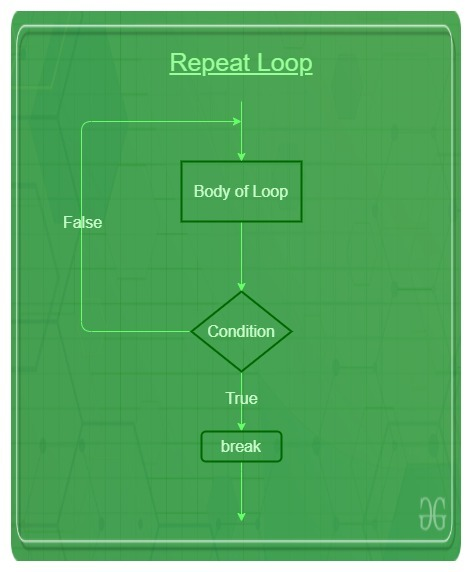GFG App
Open AppBrowser
Continue

Repeat loop in R is used to iterate over a block of code multiple number of times. And also it executes the same code again and again until a break statement is found.

Repeat loop, unlike other loops, doesn’t use a condition to exit the loop instead it looks for a break statement that executes if a condition within the loop body results to be true. An infinite loop in R can be created very easily with the help of the Repeat loop. The keyword used for the repeat loop is `'repeat'`.

Syntax:

```repeat {
commands
if(condition) {
break
}
}```

Flowchart:Example 1:

 `# R program to illustrate repeat loop ` ` `  `result <``-` `c(``"Hello World"``) ` `i <``-` `1` ` `  `# test expression  ` `repeat { ` ` `  `   ``print``(result) ` `    `  `   ``# update expression  ` `   ``i <``-` `i ``+` `1` `    `  `   ``# Breaking condition ` `   ``if``(i >``5``) { ` `      ``break` `   ``} ` `} `

Output:

``` "Hello World"
 "Hello World"
 "Hello World"
 "Hello World"
 "Hello World"```

Example 2:

 `# R program to illustrate repeat loop ` ` `  `result <``-` `1` `i <``-` `1` ` `  `# test expression  ` `repeat { ` ` `  `   ``print``(result) ` `    `  `   ``# update expression  ` `   ``i <``-` `i ``+` `1` `   ``result ``=` `result ``+` `1` ` `  `   ``# Breaking condition ` `   ``if``(i > ``5``) { ` `      ``break` `   ``} ` `} `

Output:

``` 1
 2
 3
 4
 5```

My Personal Notes arrow_drop_up